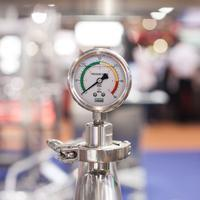# Thermal

## What to expect

The study of thermal physics covers macroscopic (whole systems) and microscopic (particles) processes.

After studying this topic, you should be able to:

• Discuss evidence for the atomic nature of materials
• Distinguish between temperature and heat
• Describe the relationship between heat added and temperature increase
• Understand why the temperature of water doesn't change when it boils
• Recall the gas laws and their associated experiments
• Explain the uses of a pV diagram
• Suggest where energy goes when work is done against friction## Key questions

#### What are the main points in kinetic theory?

Atoms and molecules are types of particle and are the smallest parts of matter. In this topic we will usually assume that the atoms or molecules are uncharged and do not interact, like a large number of very small perfectly elastic balls. Find out more.

#### What is the relationship between temperature and heat?

If two thermodynamic systems are each in thermal equilibrium with a third, then they are in thermal equilibrium with each other. In practical terms, temperature is the concentration of heat energy in a body. It dictates the overall direction in which heat energy will transfer (from hot to cold). Find out more.

#### What is latent heat?

The specific latent heat is the amount of heat required to change the state of one kilogram of a substance. Find out more.

#### What are the gas laws?

Boyle's law states that the pressure of a fixed mass of gas at constant temperature is inversely proportional to its volume. The Pressure law states that the pressure of a fixed mass of gas with constant volume is directly proportional to its absolute temperature (in Kelvin). Charles' law states that the volume of a fixed mass of gas at a constant pressure is directly proportional to its temperature in Kelvin. Find out more.

#### How do you draw a pV diagram?

PV and T can be plotted on a 3-D graph. In practice, however, we draw just p and V with the different temperatures represented by isotherms. Find out more.

•#### Thermal concepts

To understand what happens to the energy we transfer to a body when we do work against friction we need to look inside the body.

•#### Modelling gases

Because there are no forces between gas molecules, the gaseous state is the simplest to model.